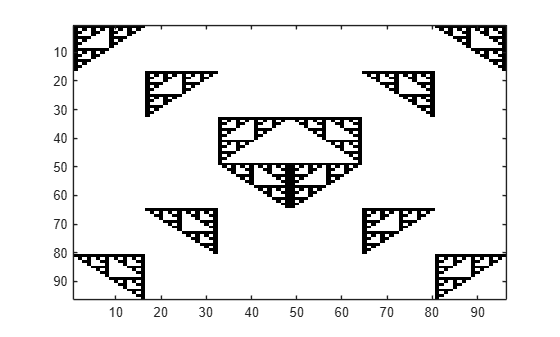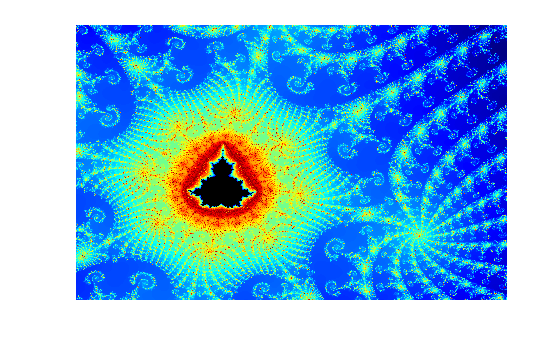# gpuArray

Array stored on GPU

## Description

A `gpuArray` object represents an array stored on the GPU. To work with `gpuArray` objects, use any GPU-enabled MATLAB® function. You can use the array for direct calculations or in CUDA kernels that execute on the GPU. For more information, see Run MATLAB Functions on a GPU.

If you want to retrieve the array from the GPU, for example when using a function that does not support `gpuArray` objects, use the `gather` function.

Note

You can load MAT files containing gpuArray data as in-memory arrays when a GPU is not available. A gpuArray loaded without a GPU is limited and you cannot use it for computations. To use a gpuArray loaded without a GPU, retrieve the contents using `gather`.

## Creation

Use `gpuArray` to convert an array in the MATLAB workspace into a `gpuArray` object. Many MATLAB functions also allow you to create `gpuArray` objects directly. For more information, see Establish Arrays on a GPU.

### Syntax

``G = gpuArray(X)``

### Description

example

````G = gpuArray(X)` copies the array `X` to the GPU and returns a `gpuArray` object. ```

### Input Arguments

expand all

Array to transfer to the GPU, specified as a numeric or logical array. The GPU device must have sufficient free memory to store the data. If `X` is already a `gpuArray` object, `gpuArray` outputs `X` unchanged.

You can also transfer sparse arrays to the GPU. `gpuArray` supports only sparse arrays of double-precision.

Example: `G = gpuArray(magic(3));`

Data Types: `single` | `double` | `int8` | `int16` | `int32` | `int64` | `uint8` | `uint16` | `uint32` | `uint64` | `logical`
Complex Number Support: Yes

## Object Functions

 `arrayfun` Apply function to each element of array on GPU `gather` Transfer distributed array or gpuArray to local workspace `pagefun` Apply function to each page of distributed array or gpuArray

There are several methods for examining the characteristics of a `gpuArray` object. Most behave like the MATLAB functions of the same name.

 `existsOnGPU` Determine if gpuArray or CUDAKernel is available on GPU `isequal` Determine array equality `isnumeric` Determine whether input is numeric array `issparse` Determine whether input is sparse `isUnderlyingType` Determine whether input has specified underlying data type `length` Length of largest array dimension `ndims` Number of array dimensions `size` Array size `underlyingType` Type of underlying data determining array behavior

Other methods for `gpuArray` objects are too numerous to list here. Most resemble and behave the same as the MATLAB functions of the same name. See Run MATLAB Functions on a GPU.

## Examples

collapse all

This example shows how to use GPU-enabled MATLAB functions to operate with gpuArrays. You can check the properties of your GPU using the `gpuDevice` function.

`gpuDevice`
```ans = CUDADevice with properties: Name: 'GeForce GTX 1080' Index: 1 ComputeCapability: '6.1' SupportsDouble: 1 DriverVersion: 10.1000 ToolkitVersion: 10.1000 MaxThreadsPerBlock: 1024 MaxShmemPerBlock: 49152 MaxThreadBlockSize: [1024 1024 64] MaxGridSize: [2.1475e+09 65535 65535] SIMDWidth: 32 TotalMemory: 8.5899e+09 AvailableMemory: 6.9012e+09 MultiprocessorCount: 20 ClockRateKHz: 1733500 ComputeMode: 'Default' GPUOverlapsTransfers: 1 KernelExecutionTimeout: 1 CanMapHostMemory: 1 DeviceSupported: 1 DeviceSelected: 1 ```

Create a row vector that repeats values from -15 to 15. To transfer it to the GPU and create a gpuArray, use the `gpuArray` function.

```X = [-15:15 0 -15:15 0 -15:15]; gpuX = gpuArray(X); whos gpuX```
``` Name Size Bytes Class Attributes gpuX 1x95 4 gpuArray ```

To operate with gpuArrays, use any GPU-enabled MATLAB function. MATLAB automatically runs calculations on the GPU. For more information, see Run MATLAB Functions on a GPU. For example, use a combination of `diag`, `expm`, `mod`, `round`, `abs`, and `fliplr`.

```gpuE = expm(diag(gpuX,-1)) * expm(diag(gpuX,1)); gpuM = mod(round(abs(gpuE)),2); gpuF = gpuM + fliplr(gpuM);```

Plot the results.

```imagesc(gpuF); colormap(flip(gray));```If you need to transfer the data back from the GPU, use `gather`. Gathering back to the CPU can be costly, and is generally not necessary unless you need to use your result with functions that do not support gpuArray.

```result = gather(gpuF); whos result```
``` Name Size Bytes Class Attributes result 96x96 73728 double ```

In general there can be differences in the results if you run the code on the CPU, due to numerical precision and algorithmic differences between GPU and CPU. Answers on CPU and GPU are both equally valid floating point approximations to the true analytical result, having been subjected to different roundoff during computation. In this example, the results are integers and `round` eliminates the roundoff errors.

This example shows how to use MATLAB functions and operators with gpuArrays to compute the integral of a function, using the Monte Carlo integration method.

Define the number of points to sample. Sample points in the domain of the function, the interval `[-1,1]` in both `x` and y coordinates, by creating random points with the `rand` function. To create a random array directly on the GPU, use the `rand` function and specify `'gpuArray'`. For more information, see Establish Arrays on a GPU.

```n = 1e6; x = 2*rand(n,1,'gpuArray')-1; y = 2*rand(n,1,'gpuArray')-1;```

Define the function to integrate, and use the Monte Carlo integration formula on it. This function approximates the value of $\pi$ by sampling points within the unit circle. Because the code uses GPU-enabled functions and operators on gpuArrays, the computations automatically run on the GPU. You can perform binary operations such as element-wise multiplication using the same syntax as MATLAB arrays use. To learn more about GPU-enabled functions, see Run MATLAB Functions on a GPU.

```f = x.^2 + y.^2 <= 1; result = 4*1/n*f'*ones(n,1,'gpuArray')```
```result = 3.1403 ```

This example shows how to use GPU-enabled MATLAB functions to compute a well-known mathematical construction: the Mandelbrot set. Check your GPU using the `gpuDevice` function.

Define the parameters. The Mandelbrot algorithm iterates over a grid of real and imaginary parts. The following code defines the number of iterations, grid size, and grid limits.

```maxIterations = 500; gridSize = 1000; xlim = [-0.748766713922161, -0.748766707771757]; ylim = [ 0.123640844894862, 0.123640851045266]; ```

You can use the `gpuArray` function to transfer data to the GPU and create a `gpuArray`, or you can create an array directly on the GPU. `gpuArray` provides GPU versions of many functions that you can use to create data arrays, such as `linspace`. For more information, see Create GPU Arrays Directly.

```x = gpuArray.linspace(xlim(1),xlim(2),gridSize); y = gpuArray.linspace(ylim(1),ylim(2),gridSize); whos x y```
``` Name Size Bytes Class Attributes x 1x1000 4 gpuArray y 1x1000 4 gpuArray ```

Many MATLAB functions support gpuArrays. When you supply a gpuArray argument to any GPU-enabled function, the function runs automatically on the GPU. For more information, see Run MATLAB Functions on a GPU. Create a complex grid for the algorithm, and create the array `count` for the results. To create this array directly on the GPU, use the `ones` function, and specify `'gpuArray'`.

```[xGrid,yGrid] = meshgrid(x,y); z0 = complex(xGrid,yGrid); count = ones(size(z0),'gpuArray');```

The following code implements the Mandelbrot algorithm using GPU-enabled functions. Because the code uses gpuArrays, the calculations happen on the GPU.

```z = z0; for n = 0:maxIterations z = z.*z + z0; inside = abs(z) <= 2; count = count + inside; end count = log(count);```

When computations are done, plot the results.

```imagesc(x,y,count) colormap([jet();flipud(jet());0 0 0]); axis off```## Tips

• If you need increased performance, or if a function is not available for GPU, `gpuArray` supports the following options:

• You can control the random number stream on the GPU using `gpurng`.

• None of the following can exceed `intmax('int32')`:

• The number of elements of a dense array.

• The number of nonzero elements of a sparse array.

• The size in any given dimension. For example, `zeros(0,3e9,'gpuArray')` is not allowed.

## Alternatives

You can also create a `gpuArray` object using some MATLAB functions by specifying a `gpuArray` output. The following table lists the available MATLAB functions that can create `gpuArray` objects directly.

 `eye(___,'gpuArray')` `rand(___,'gpuArray')` `false(___,'gpuArray')` `randi(___,'gpuArray')` `Inf(___,'gpuArray')` `randn(___,'gpuArray')` `NaN(___,'gpuArray')` `gpuArray.``colon` `ones(___,'gpuArray')` `gpuArray.``freqspace` `true(___,'gpuArray')` `gpuArray.``linspace` `zeros(___,'gpuArray')` `gpuArray.``logspace` `gpuArray.``speye`

For class-specific help on the functions with the `gpuArray` prefix, type

`help gpuArray.functionname`

where `functionname` is the name of the method. For example, to get help on `colon`, type

`help gpuArray.colon`

### Topics

Introduced in R2010b

## Support